# ImageDraw of PIL

Add line, rectangle, arc, circles to images using Pillow ImageDraw

Creating object
``PIL.ImageDraw.Draw(im, mode=None)``
im: Image to work
mode : Optional , mode to use colour values.

## Adding Lines to an image

``ImageDraw.line(xy, fill=None, width=0, joint=None)``
xy: Coordinates of the start and end points
fill: Colour of the line
width : Line width ( Integer )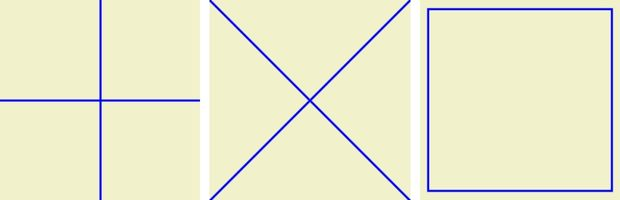We can draw a line between two coordiantes. (0,0) is the top left corner of the image.
Horizontal and vertical cross lines at center.
``````from PIL import Image, ImageDraw

path = "F:\\testing\\images\\test3.png"  # new image to create
width, height = 200, 200
img = Image.new("RGB", (width, height), color="#F1F1CC")
img1 = ImageDraw.Draw(img) # Object to draw over main image

img1.line([(0,100),(200,100)] , fill='blue',width=2,joint='curve') # Horizontal
img1.line([(100,0),(100,200)] , fill='blue',width=2,joint='curve') # Vertical
# img.save(path)
img.show()``````
Two lines crossing
``````img1.line([(0,0),(200,200)] , fill='blue',width=2,joint='curve') # cross
img1.line([(0,200),(200,0)] , fill='blue',width=2,joint='curve') # cross``````
Border in all four sides
``````img1.line([(10,10),(190,10)] , fill='blue',width=2,joint='curve') # Horizontl top
img1.line([(190,10),(190,190)] , fill='blue',width=2,joint='curve') # vertical right
img1.line([(190,190),(10,190)] , fill='blue',width=2,joint='curve') # Horizontl botton
img1.line([(10,190),(10,10)] , fill='blue',width=2,joint='curve') # vertical right``````

## Lines using For loop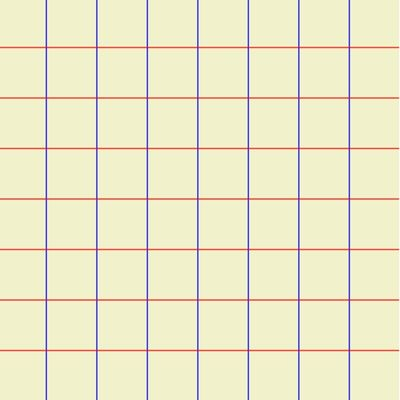``````from PIL import Image, ImageDraw

path = "E:\\testing\\images\\test3.png"  # new image to create
width, height = 400, 400
img = Image.new("RGB", (width, height), color="#F1F1CC")
img1 = ImageDraw.Draw(img) # Object to draw over main image
for i in range(0, width, 50):
img1.line([i, 0, i, height], fill="blue", width=1) # vertical
for j in range(0, height, 50):
img1.line([0, j, width, j], fill="red", width=1) # Horizontal
img.save(path)``````
We can update the thickness of the lines based on its modulus value. Here each 10th line thickness is changed.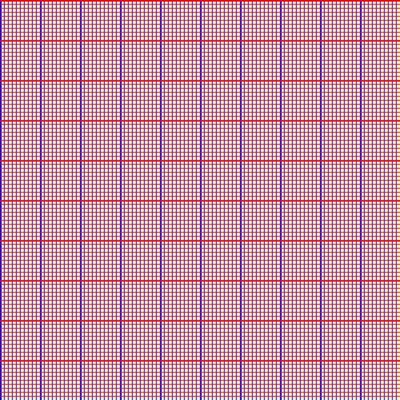``````from PIL import Image, ImageDraw
path = "E:\\testing\\images\\test3.png"  # new image to create
width, height = 500, 500
img = Image.new("RGB", (width, height), color="#F1F1CC")
img1 = ImageDraw.Draw(img) # Object to draw over main image
for i in range(0, width, 5):
if i % 50 ==0 :
img1.line([i, 0, i, height], fill="blue", width=2)
else:
img1.line([i, 0, i, height], fill="blue", width=1)

for j in range(0, height, 5):
if j% 50 ==0:
img1.line([0, j, width, j], fill="red", width=2)
else:
img1.line([0, j, width, j], fill="red", width=1)
#img.show()
img.save(path)``````

``ImageDraw.rectangle(xy, fill=None, outline=None, width=1)``
xy: Coordinates of the box
fill: Colour for filling
outline : Colour of the outline
width : Line width

Draw a border around an image with a gap of 10 from edges.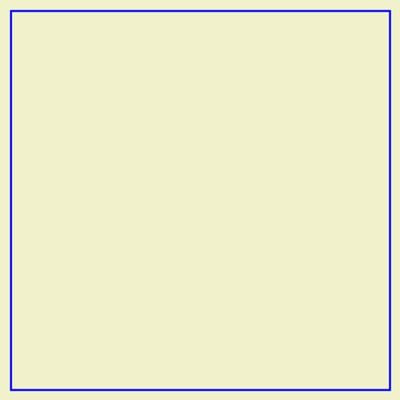``````from PIL import Image, ImageDraw
path = "F:\\testing\\images\\rect1.png"  # new image to create
width, height = 400, 400
img = Image.new("RGB", (width, height), color="#F1F1CC")
img1 = ImageDraw.Draw(img)
size=[(10,10),(width-10,height-10)] # box with coordinates
img1.rectangle(size, fill=None, outline='blue', width=2)
img.show()``````

## Concentric rectanglesHere on each step of the for loop the coordinates decreases by 10,
We have used numpy random integers to create a tuple with Random RGB numbers. Here in each step one random colour is generated.
``````from PIL import Image, ImageDraw
import numpy as np
path = "E:\\testing\\images\\rect1.png"  # new image to create
width, height = 400, 400
img = Image.new("RGB", (width, height), color="#F1F1CC")
img1 = ImageDraw.Draw(img) # create object to Draw
for i in range(0,int(width/2),10):
size=[(i,i),(width-i,height-i)] # coordinates of the rectangle
color = tuple(np.random.choice(range(255), size=3)) # random RGB colour
img1.rectangle(size, fill=None, outline=color, width=2)
img.show()
img.save(path)``````

## Adding Arc to an image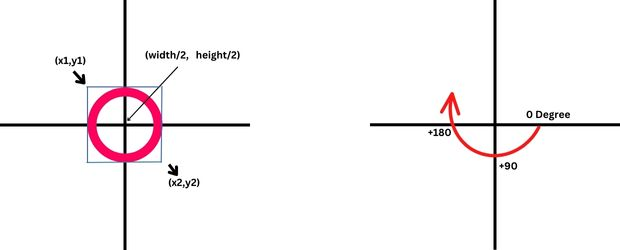``ImageDraw.arc(xy, start, end, fill=None, width=0)``
xy: Coordinates of the box
start: Starting angle of the arc. Starts from 3 o’clock, increase clockwise
end: Ending angle in degree
fill: Colour for filling
width : Line width

## Circle at Center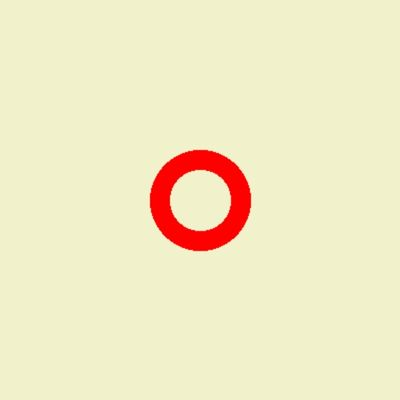``````from PIL import Image, ImageDraw
path = "E:\\testing\\images\\arc1.png"  # new image to create
width, height = 400, 400
img = Image.new("RGB", (width, height), color="#F1F1CC")
img1 = ImageDraw.Draw(img) # create object to Draw
size=[(width/2)-50,(height/2)-50,(width/2)+50,(height/2)+50]
img1.arc(size,0,360, fill='red', width=20)
img.show()
img.save(path)``````

## Concentric Circles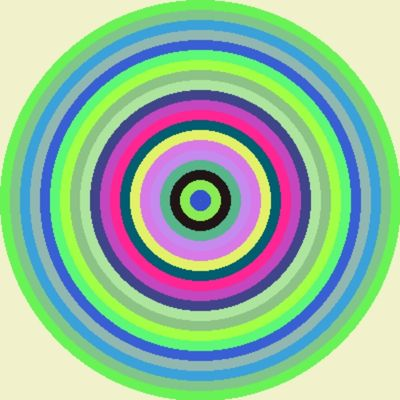Here the same concept of concentric rectangles ( above explained ) is used.
``````from PIL import Image, ImageDraw
import numpy as np
path = "C:\\testing\\images\\arc1.png"  # new image to create
width, height = 400, 400
img = Image.new("RGB", (width, height), color="#F1F1CC")
img1 = ImageDraw.Draw(img) # create object to Draw
for i in range(0,int(width/2),10):
size=[(i,i),(width-i,height-i)] # box around arc
color = tuple(np.random.choice(range(255), size=3)) # ranom RGB value
img1.arc(size,0,360, fill=color, width=11)
img.show()
img.save(path)``````

## Half ArcHere start angle is 180 degree and ending angle is 360 degree.
``````from PIL import Image, ImageDraw
path = "E:\\testing\\images\\arc1.png"  # new image to create
width, height = 400, 400
img = Image.new("RGB", (width, height), color="#F1F1CC")
img1 = ImageDraw.Draw(img) # create object to Draw
size=[(width/2)-190,(height/2)-190,(width/2)+190,(height/2)+190]
img1.arc(size,180,360, fill='red', width=50)
img.show()
img.save(path)``````

Subscribe to our YouTube Channel here

## Subscribe

* indicates required
Subscribe to plus2netplus2net.com